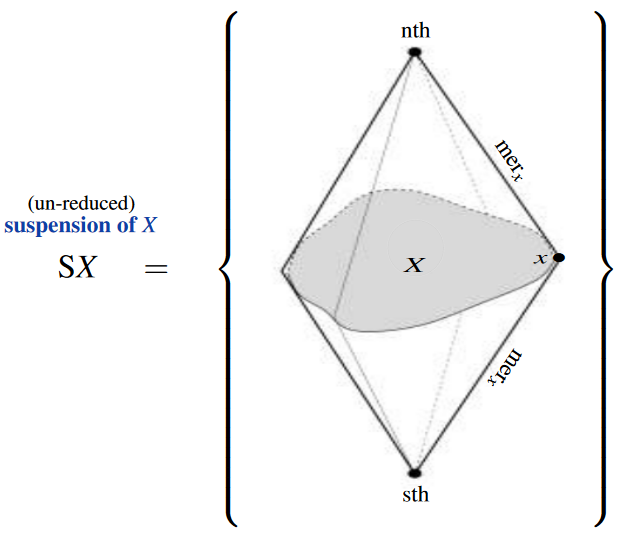Contents

# Contents

## Idea

In homotopy type theory, a suspension type of any type $X$ is the pushout type of the terminal function $X \to \ast$ (to the unit type) along itself, hence the pushout of the span $\ast \longleftarrow X \longrightarrow \ast$.

The categorical semantics is given by suspension objects in homotopy theory (and hence by suspension of topological spaces in, say, the classical model structure on topological spaces).

## Definition

As a higher inductive type, the (unreduced) suspension $\mathrm{S} X$ of a type $X$ is generated by

1. A term $\mathrm{nth} \colon \mathrm{S} X$

2. a term $\mathrm{sth} \colon \mathrm{S} X$

3. A function $mer \colon X \to Id_{\mathrm{S}X}\big(nth,\, sth\big)$

(to the identity type of $\mathrm{S}X$).The resulting inference rules are the specialization of those of pushout types (see there).

In Coq pseudocode this becomes

Inductive Suspension (X \colon Type) : Type
| nth : Suspension X
| sth : Suspension X
| mer : X -> Id Suspension X nth sth

This says that the type is dependent on the type $X$ and inductive constructed from two terms in the suspension, whose interpretation is as the north and south poles of the suspension, together with a term in the function type from $X$ to the identity type of paths between these two terms, representing the meridians from the north to the south pole.

## Examples

• The two-valued type $\mathbf{2}$ is the suspension type of the empty type $\mathbf{0}$.

• The interval type $I$ is the suspension type of the unit type $\mathbf{1}$.

• The circle type $S^1$ is the suspension type of $\mathbf{2}$.

• The homotopical disk type $G_2$ is the suspension type of $I$.

• Homotopical $n$-sphere types of dimension $n:\mathbb{N}$, $S^n$, are suspension types of $S^{n-1}$.

• Homotopical $n$-globe types of dimension $n:\mathbb{N}$, $G_n$, are suspension types of $G_{n-1}$.